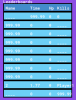# SOLVEDSorting arrays

#### Darnok

##### Member
hey i want to save leaderboards in my game i first made them with ds grids but got told that they cant be saved so good and i sould redo it so it can be saved as a JSON for that arrays would work i got told so i remade my grids in arrays thats good and all and works but before i used the ds_grid_sort i know there is also a array_sort but i dont know how to use it like a grid sort i dont want to only sort single values i have to sort 4 valus (name,time,hp,kills) to sort i want to use the time value

old code for adding a score
GML:
``````function add_to_leaderboard(lb_Room, lb_Name, lb_Time, lb_hp, lb_kills ){

for(i=0;i<ds_grid_width(global.grid_levels);i++;)
{
if(ds_grid_get(global.grid_levels,i,1) = lb_Room)
{

global.grid_levels[# i, 0][# 0,10] = lb_Name;
global.grid_levels[# i, 0][# 1,10] = lb_Time;
global.grid_levels[# i, 0][# 2,10] = lb_hp;
global.grid_levels[# i, 0][# 3,10] = lb_kills;

ds_grid_sort(global.grid_levels[# i, 0],1,true)

}
}

}``````
GML:
``````global.array_levels = array_create(100)//levels
for(i=0;i<100;i++;)
{
for(j=0;j<3;j++;)
{
global.array_levels[i][j] = array_create(4)//name,time,hp,kills
for(k=0;k<4;k++;)
{
global.array_levels[i][j][k] = array_create(11)//rank 1-11
}
}
}``````
after we got a solution for that problem i will probably still need help with saving and loading it but letz solve this firstLast edited:

#### Ommn

##### Member
for sorting use array_sort:
GML:
``array_sort(global.array_levels[i][j], function(a,b){return a-b;})``
for save you can save array as string in text file:
GML:
``````var __file=file_text_open_write("save.txt")
file_text_write_string(__file,string(global.array_levels))
file_text_close(__file)``````
GML:
``````var __file=file_text_open_read("save.txt")
file_text_close(__file)``````

#### Darnok

##### Member
thanks a lot so far the saving works but i didnt understand what you did at that sort there

i have a 4d array right now and the number were i want to sort (smallest = rank 1) is at
GML:
``global.array_levels[i]``
but i dont only want to sort the numbers but also the things that are with them so if i sort by time the name,hp,kills should just move with it

i would love if you can explain the sort part a bit better i this part in peticular
GML:
``function(a,b){return a-b;}``
thx#### TailBit

##### Member
I would recommend you to switch these:

GML:
``````        global.array_levels[i][j] = array_create(4)//name,time,hp,kills
for(k=0;k<4;k++;)
{
global.array_levels[i][j][k] = array_create(11)//rank 1-11
}``````
so that they are:

GML:
``````        global.array_levels[i][j] = array_create(11)//rank 1-11
for(k=0;k<11;k++)
{
global.array_levels[i][j][k] = array_create(4)//name,time,hp,kills
}``````
because the sort function can sort one list .. but it can't sort 4 at once .. if all the stats are in each their ranking position, then they can be moved as a unit .. but if name,time and such each got their list and they all have to be shuffled together, then you can't use the array_sort function

Time is also index 1:
`array_sort(global.array_levels[i][j], function(a,b){return a-b;})`
the sort will take 2 positions in the array and feed it to the function so a might be ["bob",23,34,21] which could be index 0 in the array, which is compared to index 1 ["Tim",22,43,56] .. so they will be feed into the function as a and b and compared .. here we take their time against them .. if the value returned is positive then one gets sorted on top, if negative then the other one get sorted on top of the 2

#### Darnok

##### Member
ok so i think i still did understand you wrong i now have to code for the sort

GML:
``````for(i=0;i<100;i++;)
{
if(global.array_levels[i] = lb_room)
{
global.array_levels[i] = lb_name;
global.array_levels[i] = lb_time;
global.array_levels[i] = lb_hp;
global.array_levels[i] = lb_kills;

for(j=9;j>0;j--;)
{
array_sort(global.array_levels[i][j],  lb_sort(global.array_levels[i][j],global.array_levels[i][j-1]))
}

}
}``````
GML:
``````function lb_sort(a,b){

{return a-b;}
}``````
but it dose not work how indendet it moves the values to the left not up / downwhat did i do wrong ? thx

#### TailBit

##### Member
if you made it into a script then don't fill it in .. you are just telling the array sort what function it should use for comparing .. it compare them against eachother using that function you provide, so you didn't really have to change anything there (you just made it a global function if you put it into a script):
`array_sort(global.array_levels[i], lb_sort )`

the score array is in `global.array_levels[i]` right?
no need for the j loop there

#### Darnok

##### Member
in `global.array_levels[i]` is now the array with the 11 ranks and in there the array with the 4 valus name,time,hp,kills
but if i do
GML:
``````    for(i=0;i<100;i++;)
{
if(global.array_levels[i] = lb_room)
{
global.array_levels[i] = lb_name;
global.array_levels[i] = lb_time;
global.array_levels[i] = lb_hp;
global.array_levels[i] = lb_kills;
show_debug_message("sorting array")

array_sort(global.array_levels[i],lb_sort)

show_debug_message("array sorted")

}
}``````
now nothing happens the code runs but after a bit bug finding i saw that the function is not called / runned you have a idea why ?
GML:
``````function lb_sort(a,b){

show_debug_message("im a sort")
{return a-b;}
}``````
it showes "sorting array" and then "array sorted" but not the "im a sort"

thx#### TailBit

##### Member
try change it back to:
`array_sort(global.array_levels[i],function(a,b){ return a-b; });`
maybe it can't use global functions?

#### Darnok

##### Member
ok that was it thx•TailBit